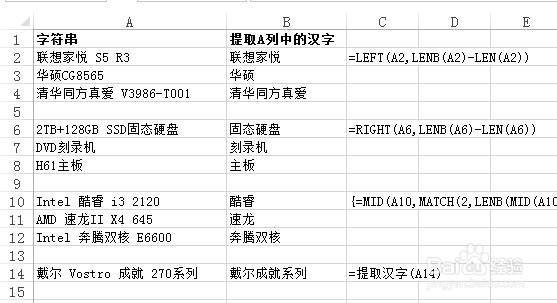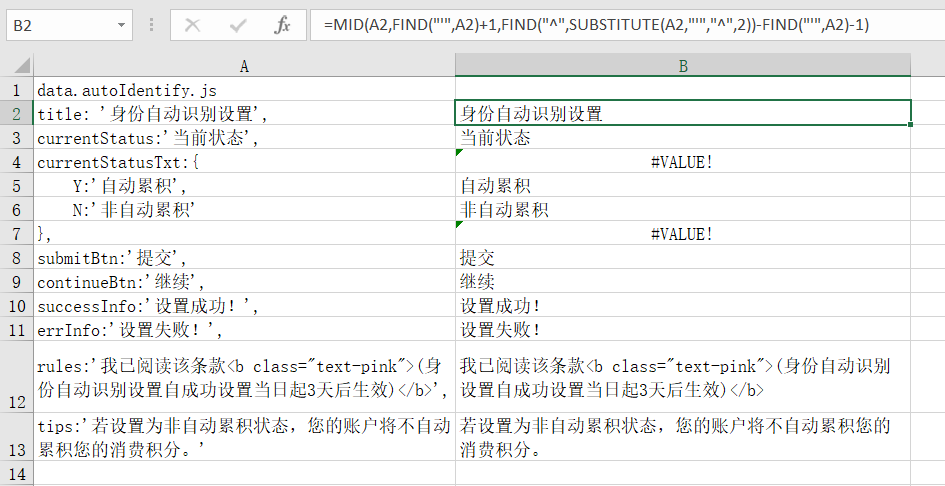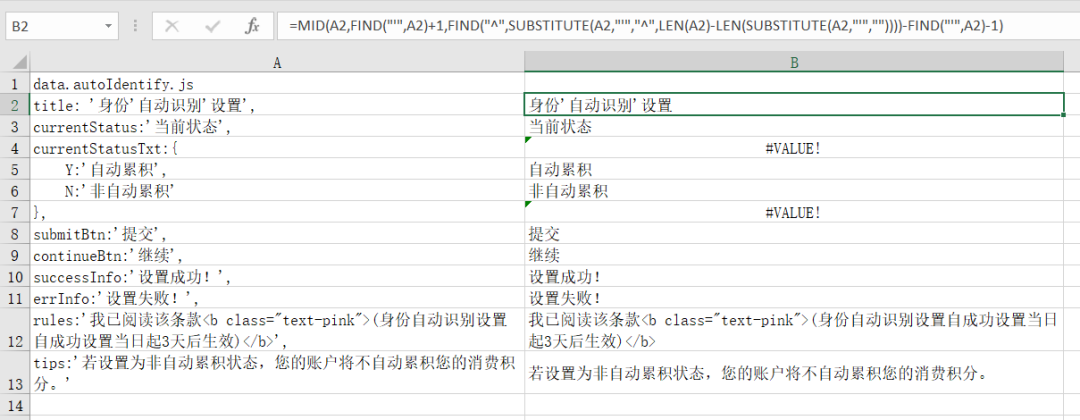# 用公式提取Excel单元格中的汉字

### 一、用公式提取Excel单元格中的汉字=LEFT(A2,LENB(A2)-LEN(A2))

=RIGHT(A6,LENB(A6)-LEN(A6))

=MID(A10,MATCH(2,LENB(MID(A10,ROW(INDIRECT(“1:”&LEN(A10))),1)),),LENB(A10)-LEN(A10))

1. 按Alt+F11，打开VBA编辑器，单击菜单“插入→模块”，在代码窗口中输入粘贴下面的代码：

Function 提取汉字(sString As String) As String
Dim regEx As Object
Set regEx = CreateObject(“VBScript.RegExp”)
With regEx
‘搜索整个字符串
.Global = True
‘匹配非汉字
.Pattern = “[^\u4e00-\u9fa5]”
‘将字符串中的非汉字替换为空

End With
Set regEx = Nothing
End Function

2. 返回Excel工作表界面，在B14单元格中输入公式：

=提取汉字(A14)

### 二、用公式提取引号（某2个相同字符）之间的内容=MID(A2,FIND(“‘”,A2)+1,FIND(“^”,SUBSTITUTE(A2,”‘”,”^”,LEN(A2)-LEN(SUBSTITUTE(A2,”‘”,””))))-FIND(“‘”,A2)-1)

=FIND(“这个不重复就行”,SUBSTITUTE(A1,”-“,” 这个不重复就行”,LEN(A1)-LEN(SUBSTITUTE(A1,”-“,””))))

=find(“这个不重复就行”,substitute(A1,”\”,”这个不重复就行”,4))

=FIND(“”””,E11)

Excel

## Excel统计行内空白单元格的个数

2018-3-25 15:11:36

SQL

## 数据库基础知识总结

2018-3-22 0:29:50

0 条回复 A文章作者 M管理员
暂无讨论，说说你的看法吧